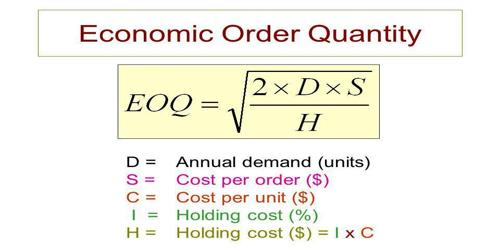Accounting

# Economic Order QuantityEconomic order quantity (EOQ) is the order quantity.bf inventory that minimizes the total cost of inventory management. It is a measurement used in the field of Operations, Logistics, and Supply Management.

Two most important categories of inventory costs are ordering costs and carrying costs. Ordering costs are costs that are incurred on obtaining additional inventories. They include costs incurred on communicating the order, transportation cost, etc. Carrying costs represent the costs incurred on holding inventory in hand. They include the opportunity cost of money held up in inventories, storage costs, spoilage costs, etc.

Ordering costs and carrying costs are quite opposite to each other. If we need to minimize carrying costs we have to place a small order which increases the ordering costs. If we want to minimize our ordering costs we have to place a few orders in a year and this requires placing large orders which in turn increases the total carrying costs for the period. This is done to minimize variable inventory costs, and the equation for EOQ takes into account storage, ordering costs and shortage costs.

Total inventory costs = Ordering Costs + Holding Costs

By taking the first derivative of the function we find the following equation for minimum cost –

EOQ = √[(2 x Quantity x Cost Per Order) / Carrying Cost Per Order]

EOQ formula can be customized to find out dissimilar production levels or order interval lengths, and corporations with large supply chains and high variable costs use an algorithm in their computer software to find out EOQ.